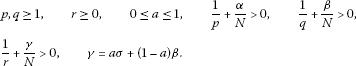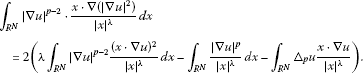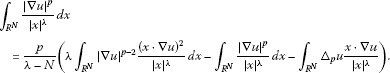# A note on a class of Hardy-Rellich type inequalities

## Abstract

In this note we provide simple and short proofs for a class of Hardy-Rellich type inequalities with the best constant, which extends some recent results.

MSC:26D15, 35A23.

## 1 Introduction

It is well known that Hardy’s inequality and its generalizations play important roles in many areas of mathematics. The classical Hardy inequality is given by, for $N\ge 3$,

${\int }_{{R}^{N}}{|\mathrm{\nabla }u\left(x\right)|}^{2}\phantom{\rule{0.2em}{0ex}}dx\ge {\left(\frac{N-2}{2}\right)}^{2}{\int }_{{R}^{N}}\frac{{|u\left(x\right)|}^{2}}{{|x|}^{2}}\phantom{\rule{0.2em}{0ex}}dx,$
(1.1)

where $u\in {C}_{0}^{\mathrm{\infty }}\left({R}^{N}\right)$, the constant ${\left(\frac{N-2}{2}\right)}^{2}$ is optimal and not attained.

Recently there has been a considerable interest in studying the Hardy-type and Rellich-type inequalities. See, for example, . In  Caffarelli, Kohn and Nirenberg proved a rather general interpolation inequality with weights. That is the following so-called Caffarelli-Kohn-Nirenberg inequality. For any $u\in {C}_{0}^{\mathrm{\infty }}\left({R}^{N}\right)$, there exists $C>0$ such that

${\parallel {|x|}^{\gamma }u\parallel }_{{L}^{r}}\le C{\parallel {|x|}^{\alpha }|\mathrm{\nabla }u|\parallel }_{{L}^{p}}^{a}\cdot {\parallel {|x|}^{\beta }u\parallel }_{{L}^{q}}^{1-a},$
(1.2)

where

$\frac{1}{r}+\frac{\gamma }{N}=a\left(\frac{1}{p}+\frac{\alpha -1}{N}\right)+\left(1-a\right)\left(\frac{1}{q}+\frac{\beta }{N}\right)$

andIn  Costa proved the following ${L}^{2}$-case version for a class of Caffarelli-Kohn-Nirenberg inequalities with a sharp constant by an elementary method. For all $a,b\in R$ and $u\in {C}_{0}^{\mathrm{\infty }}\left({R}^{N}\mathrm{\setminus }\left\{0\right\}\right)$,

$\stackrel{ˆ}{C}{\int }_{{R}^{N}}\frac{{|u|}^{2}}{{|x|}^{a+b+1}}\phantom{\rule{0.2em}{0ex}}dx\le {\left({\int }_{{R}^{N}}\frac{{|u|}^{2}}{{|x|}^{2a}}\phantom{\rule{0.2em}{0ex}}dx\right)}^{\frac{1}{2}}{\left({\int }_{{R}^{N}}\frac{{|\mathrm{\nabla }u|}^{2}}{{|x|}^{2b}}\phantom{\rule{0.2em}{0ex}}dx\right)}^{\frac{1}{2}},$
(1.3)

where the constant $\stackrel{ˆ}{C}=\stackrel{ˆ}{C}\left(a,b\right):=\frac{|N-\left(a+b+1\right)|}{2}$ is sharp.

On the other hand, the Rellich inequality is a generalization of the Hardy inequality to second-order derivatives, and the classical Rellich inequality in ${R}^{N}$ states that for $N\ge 5$ and $u\in {C}_{0}^{\mathrm{\infty }}\left({R}^{N}\setminus \left\{0\right\}\right)$,

${\int }_{{R}^{N}}{|\mathrm{\Delta }u\left(x\right)|}^{2}\phantom{\rule{0.2em}{0ex}}dx\ge {\left(\frac{N\left(N-4\right)}{4}\right)}^{2}{\int }_{{R}^{N}}\frac{{|u\left(x\right)|}^{2}}{{|x|}^{4}}\phantom{\rule{0.2em}{0ex}}dx.$
(1.4)

The constant $\frac{{N}^{2}{\left(N-4\right)}^{2}}{16}$ is sharp and never achieved. In  Tetikas and Zographopoulos obtained a corresponding stronger versions of the Rellich inequality which reads

${\left(\frac{N}{2}\right)}^{2}{\int }_{{R}^{N}}\frac{{|\mathrm{\nabla }u|}^{2}}{{|x|}^{2}}\phantom{\rule{0.2em}{0ex}}dx\le {\int }_{{R}^{N}}{|\mathrm{△}u|}^{2}\phantom{\rule{0.2em}{0ex}}dx$
(1.5)

for all $u\in {C}_{0}^{\mathrm{\infty }}$ and $N\ge 3$. In  Costa obtained a new class of Hardy-Rellich type inequalities which contain (1.5) as a special case. If $a+b+3\le N$, then

$\stackrel{ˆ}{C}{\int }_{{R}^{N}}\frac{{|\mathrm{\nabla }u|}^{2}}{{|x|}^{a+b+1}}\phantom{\rule{0.2em}{0ex}}dx\le {\left({\int }_{{R}^{N}}\frac{{|\mathrm{△}u|}^{2}}{{|x|}^{2b}}\phantom{\rule{0.2em}{0ex}}dx\right)}^{\frac{1}{2}}{\left({\int }_{{R}^{N}}\frac{{|\mathrm{\nabla }u|}^{2}}{{|x|}^{2a}}\phantom{\rule{0.2em}{0ex}}dx\right)}^{\frac{1}{2}},$
(1.6)

where the constant $\stackrel{ˆ}{C}=\stackrel{ˆ}{C}\left(a,b\right):=|\frac{N+a+b-1}{2}|$ is sharp.

The goal of this paper is to extend the above (1.3) and (1.6) to the general ${L}^{p}$ case for $1 by a different and direct approach.

## 2 Main results

In this section, we will give the proof of the main theorems.

Theorem 1 For all $a,b\in R$ and $u\in {C}_{0}^{\mathrm{\infty }}\left({R}^{N}\setminus \left\{0\right\}\right)$, one has

$C{\int }_{{R}^{N}}\frac{{|u|}^{p}}{{|x|}^{a+b+1}}\phantom{\rule{0.2em}{0ex}}dx\le {\left({\int }_{{R}^{N}}\frac{{|\mathrm{\nabla }u|}^{p}}{{|x|}^{ap}}\phantom{\rule{0.2em}{0ex}}dx\right)}^{\frac{1}{p}}{\left({\int }_{{R}^{N}}\frac{{|u|}^{p}}{{|x|}^{b\frac{p}{p-1}}}\phantom{\rule{0.2em}{0ex}}dx\right)}^{\frac{p-1}{p}},$
(2.1)

where $1 and the constant $C=|\frac{N-\left(a+b+1\right)}{p}|$ is sharp.

Proof Let $u\in {C}_{0}^{\mathrm{\infty }}\left({R}^{N}\setminus \left\{0\right\}\right)$, $a,b\in R$ and $\lambda =a+b+1$. By integration by parts and the Hölder inequality, one has

$\begin{array}{rcl}{\int }_{{R}^{N}}\frac{{|u|}^{p}}{{|x|}^{\lambda }}\phantom{\rule{0.2em}{0ex}}dx& =& \frac{1}{N-\lambda }{\int }_{{R}^{N}}{|u|}^{p}div\left(\frac{x}{{|x|}^{\lambda }}\right)\phantom{\rule{0.2em}{0ex}}dx\\ =& -\frac{1}{N-\lambda }{\int }_{{R}^{N}}pu{|u|}^{p-2}\frac{x\cdot \mathrm{\nabla }u}{{|x|}^{\lambda }}\phantom{\rule{0.2em}{0ex}}dx\\ \le & |\frac{-p}{N-\lambda }|{\int }_{{R}^{N}}\frac{|x\cdot \mathrm{\nabla }u|}{{|x|}^{\lambda }}{|u|}^{p-1}\phantom{\rule{0.2em}{0ex}}dx\\ \le & |\frac{p}{N-\lambda }|{\int }_{{R}^{N}}\frac{|\mathrm{\nabla }u|{|u|}^{p-1}}{{|x|}^{a+b}}\phantom{\rule{0.2em}{0ex}}dx\\ \le & |\frac{p}{N-\lambda }|{\left({\int }_{{R}^{N}}\frac{{|\mathrm{\nabla }u|}^{p}}{{|x|}^{ap}}\phantom{\rule{0.2em}{0ex}}dx\right)}^{\frac{1}{p}}{\left({\int }_{{R}^{N}}\frac{{|u|}^{p}}{{|x|}^{b\frac{p}{p-1}}}\phantom{\rule{0.2em}{0ex}}dx\right)}^{\frac{p-1}{p}}.\end{array}$

Then

$|\frac{N-\lambda }{p}|{\int }_{{R}^{N}}\frac{{|u|}^{p}}{{|x|}^{\lambda }}\phantom{\rule{0.2em}{0ex}}dx\le {\left({\int }_{{R}^{N}}\frac{{|\mathrm{\nabla }u|}^{p}}{{|x|}^{ap}}\phantom{\rule{0.2em}{0ex}}dx\right)}^{\frac{1}{p}}{\left({\int }_{{R}^{N}}\frac{{|u|}^{p}}{{|x|}^{b\frac{p}{p-1}}}\phantom{\rule{0.2em}{0ex}}dx\right)}^{\frac{p-1}{p}}.$
(2.2)

It remains to show the sharpness of the constant. By the condition with equality in the Hölder inequality, we consider the following family of functions:

and

where ${C}_{\epsilon }$ is a positive number sequence converging to $|\frac{N-\left(a+b+1\right)}{p}|$ as $\epsilon \to 0$. By direct computation and the limit process, we know the constant $\frac{|N-\left(a+b+1\right)|}{p}$ is sharp. □

Remark 1 When $p=2$, the inequality (2.1) covers the inequality (2.4) in .

Remark 2 When $a=0$, $b=p-1$, the inequality (2.1) is the classical ${L}^{p}$ Hardy inequality:

${\left(\frac{N-p}{p}\right)}^{p}{\int }_{{R}^{N}}\frac{{|u|}^{p}}{{|x|}^{p}}\phantom{\rule{0.2em}{0ex}}dx\le {\int }_{{R}^{N}}{|\mathrm{\nabla }u|}^{p}\phantom{\rule{0.2em}{0ex}}dx.$
(2.3)

When we take special values for a, b, the following corollary holds.

Corollary 1 (i) When $b=\left(a+1\right)\left(p-1\right)$, the inequality (2.1) is just the weighted Hardy inequality:

$|\frac{N-p\left(a+1\right)}{p}{|}^{p}{\int }_{{R}^{N}}\frac{{|u|}^{p}}{{|x|}^{\left(a+1\right)p}}\phantom{\rule{0.2em}{0ex}}dx\le {\int }_{{R}^{N}}\frac{{|\mathrm{\nabla }u|}^{p}}{{|x|}^{ap}}\phantom{\rule{0.2em}{0ex}}dx.$
(2.4)
1. (ii)

When $a+b+1=ap$, according to the inequality (2.1), we have

$|\frac{N-ap}{p}|{\int }_{{R}^{N}}\frac{{|u|}^{p}}{{|x|}^{ap}}\phantom{\rule{0.2em}{0ex}}dx\le {\left({\int }_{{R}^{N}}\frac{{|\mathrm{\nabla }u|}^{p}}{{|x|}^{ap}}\phantom{\rule{0.2em}{0ex}}dx\right)}^{\frac{1}{p}}{\left({\int }_{{R}^{N}}\frac{{|u|}^{p}}{{|x|}^{ap-\frac{p}{p-1}}}\phantom{\rule{0.2em}{0ex}}dx\right)}^{\frac{p-1}{p}}.$
(2.5)
2. (iii)

When $a=-p$ and $a+b+1=0$, we obtain the inequality

$\frac{N}{p}{\int }_{{R}^{N}}{|u|}^{p}\phantom{\rule{0.2em}{0ex}}dx\le {\left({\int }_{{R}^{N}}{|\mathrm{\nabla }u|}^{p}{|x|}^{{p}^{2}}\phantom{\rule{0.2em}{0ex}}dx\right)}^{\frac{1}{p}}{\left({\int }_{{R}^{N}}\frac{{|u|}^{p}}{{|x|}^{p}}\phantom{\rule{0.2em}{0ex}}dx\right)}^{\frac{p-1}{p}}.$
(2.6)

By a similar method, we can prove the following ${L}^{p}$ case Hardy-Rellich type inequality.

Theorem 2 Let $1, $\frac{p-N}{p-1}\le a+b+1\le 0$. Then, for any $u\in {C}_{0}^{\mathrm{\infty }}\left({R}^{N}\setminus \left\{0\right\}\right)$, the following holds:

$\stackrel{ˆ}{C}{\int }_{{R}^{N}}\frac{{|\mathrm{\nabla }u|}^{p}}{{|x|}^{a+b+1}}\phantom{\rule{0.2em}{0ex}}dx\le {\left({\int }_{{R}^{N}}\frac{{|{\mathrm{△}}_{p}u|}^{p}}{{|x|}^{ap}}\phantom{\rule{0.2em}{0ex}}dx\right)}^{\frac{1}{p}}{\left({\int }_{{R}^{N}}\frac{{|\mathrm{\nabla }u|}^{q}}{{|x|}^{bq}}\phantom{\rule{0.2em}{0ex}}dx\right)}^{\frac{1}{q}},$
(2.7)

where $\frac{1}{p}+\frac{1}{q}=1$, $\stackrel{ˆ}{C}=\left(\frac{N-p+\left(p-1\right)\left(a+b+1\right)}{p}\right)$ and ${\mathrm{△}}_{p}u=div\left({|\mathrm{\nabla }u|}^{p-2}\mathrm{\nabla }u\right)$ is the p-Laplacian operator.

Proof Set $\lambda =a+b+1$, it is easy to see

$\begin{array}{rl}{\int }_{{R}^{N}}\frac{{|\mathrm{\nabla }u|}^{p}}{{|x|}^{\lambda }}\phantom{\rule{0.2em}{0ex}}dx& =\frac{1}{N-\lambda }{\int }_{{R}^{N}}{|\mathrm{\nabla }u|}^{p}div\left(\frac{x}{{|x|}^{\lambda }}\right)\phantom{\rule{0.2em}{0ex}}dx\\ =-\frac{1}{N-\lambda }{\int }_{{R}^{N}}\frac{p}{2}{|\mathrm{\nabla }u|}^{p-2}\frac{x}{{|x|}^{\lambda }}\cdot \mathrm{\nabla }\left({|\mathrm{\nabla }u|}^{2}\right)\phantom{\rule{0.2em}{0ex}}dx\\ =\frac{p}{2\left(\lambda -N\right)}{\int }_{{R}^{N}}{|\mathrm{\nabla }u|}^{p-2}\frac{x\cdot \mathrm{\nabla }\left({|\mathrm{\nabla }u|}^{2}\right)}{{|x|}^{\lambda }}\phantom{\rule{0.2em}{0ex}}dx.\end{array}$
(2.8)

On the other hand,

$\begin{array}{rcl}{\int }_{{R}^{N}}{\mathrm{△}}_{p}u\frac{x\cdot \mathrm{\nabla }u}{{|x|}^{\lambda }}\phantom{\rule{0.2em}{0ex}}dx& =& {\int }_{{R}^{N}}div\left({|\mathrm{\nabla }u|}^{p-2}\mathrm{\nabla }u\right)\frac{x\cdot \mathrm{\nabla }u}{{|x|}^{\lambda }}\phantom{\rule{0.2em}{0ex}}dx\\ =& -{\int }_{{R}^{N}}{|\mathrm{\nabla }u|}^{p-2}\mathrm{\nabla }u\cdot \mathrm{\nabla }\left(\frac{x\cdot \mathrm{\nabla }u}{{|x|}^{\lambda }}\right)\phantom{\rule{0.2em}{0ex}}dx\\ =& -{\int }_{{R}^{N}}{|\mathrm{\nabla }u|}^{p-2}\left(\frac{{|\mathrm{\nabla }u|}^{2}}{{|x|}^{\lambda }}+\frac{\frac{1}{2}x\cdot \mathrm{\nabla }\left({|\mathrm{\nabla }u|}^{2}\right)}{{|x|}^{\lambda }}-\lambda \frac{{\left(x\cdot \mathrm{\nabla }u\right)}^{2}}{{|x|}^{\lambda +2}}\right)\phantom{\rule{0.2em}{0ex}}dx,\end{array}$

which means(2.9)

Then, we can deduce from (2.8) and (2.9)(2.10)

That is,

$\frac{N-p-\lambda }{p}{\int }_{{R}^{N}}\frac{{|\mathrm{\nabla }u|}^{p}}{{|x|}^{\lambda }}\phantom{\rule{0.2em}{0ex}}dx+\lambda {\int }_{{R}^{N}}{|\mathrm{\nabla }u|}^{p-2}\frac{{\left(x\cdot \mathrm{\nabla }u\right)}^{2}}{{|x|}^{\lambda +2}}\phantom{\rule{0.2em}{0ex}}dx={\int }_{{R}^{N}}{\mathrm{△}}_{p}u\frac{x\cdot \mathrm{\nabla }u}{{|x|}^{\lambda }}\phantom{\rule{0.2em}{0ex}}dx.$
(2.11)

By the Hölder inequality,

${\int }_{{R}^{N}}{\mathrm{△}}_{p}u\frac{x\cdot \mathrm{\nabla }u}{{|x|}^{\lambda +2}}\phantom{\rule{0.2em}{0ex}}dx\le {\left({\int }_{{R}^{N}}\frac{{|{\mathrm{△}}_{p}u|}^{q}}{{|x|}^{aq}}\right)}^{\frac{1}{q}}{\left({\int }_{{R}^{N}}\frac{{|\mathrm{\nabla }u|}^{p}}{{|x|}^{bp}}\right)}^{\frac{1}{p}},$
(2.12)

note that $\frac{p-N}{p-1}\le \lambda \le 0$. Thus

$\frac{N-p+\left(p-1\right)\lambda }{p}{\int }_{{R}^{N}}\frac{{|\mathrm{\nabla }u|}^{p}}{{|x|}^{\lambda }}\phantom{\rule{0.2em}{0ex}}dx\le {\left({\int }_{{R}^{N}}\frac{{|{\mathrm{△}}_{p}u|}^{p}}{{|x|}^{ap}}\right)}^{\frac{1}{p}}{\left({\int }_{{R}^{N}}\frac{{|\mathrm{\nabla }u|}^{q}}{{|x|}^{bq}}\right)}^{\frac{1}{q}}.$
(2.13)

We mention that we do not know whether the constant $\left(\frac{N-p+\left(p-1\right)\left(a+b+1\right)}{p}\right)$ in (2.7) is optimal or not. □

Corollary 2 When $a+b+1=0$, we have the following inequalities:

1. (i)

when $a=-1$, $b=0$, the inequality (2.7) is equivalent to the inequality

${\left(\frac{N-p}{p}\right)}^{p}{\int }_{{R}^{N}}{|\mathrm{\nabla }u|}^{p}\phantom{\rule{0.2em}{0ex}}dx\le {\int }_{{R}^{N}}{|{\mathrm{△}}_{p}u|}^{p}{|x|}^{p}\phantom{\rule{0.2em}{0ex}}dx.$
(2.14)
2. (ii)

When $a=1$, $b=-2$, we obtain the inequality

$\left(\frac{N-p}{p}\right){\int }_{{R}^{N}}{|\mathrm{\nabla }u|}^{p}\phantom{\rule{0.2em}{0ex}}dx\le {\left({\int }_{{R}^{N}}\frac{{|{\mathrm{△}}_{p}u|}^{p}}{{|x|}^{p}}\phantom{\rule{0.2em}{0ex}}dx\right)}^{\frac{1}{p}}{\left({\int }_{{R}^{N}}{|\mathrm{\nabla }u|}^{q}{|x|}^{2q}\phantom{\rule{0.2em}{0ex}}dx\right)}^{\frac{1}{q}}.$
(2.15)
3. (iii)

When $a=0$, $b=-1$, we get

$\left(\frac{N-p}{p}\right){\int }_{{R}^{N}}{|\mathrm{\nabla }u|}^{p}\phantom{\rule{0.2em}{0ex}}dx\le {\left({\int }_{{R}^{N}}{|{\mathrm{△}}_{p}u|}^{p}\phantom{\rule{0.2em}{0ex}}dx\right)}^{\frac{1}{p}}{\left({\int }_{{R}^{N}}{|\mathrm{\nabla }u|}^{q}{|x|}^{q}\phantom{\rule{0.2em}{0ex}}dx\right)}^{\frac{1}{q}}.$
(2.16)

## References

1. Adimurthi AS: Role of the fundamental solution in Hardy-Sobolev type inequalities. Proc. R. Soc. Edinb., Sect. A 2006, 136: 1111–1130. 10.1017/S030821050000490X

2. Garofalo N, Lanconelli E: Frequency functions on the Heisenberg group, the uncertainty principle and unique continuation. Ann. Inst. Fourier (Grenoble) 1990, 40: 313–356. 10.5802/aif.1215

3. Goldstein JA, Kombe I: Nonlinear degenerate parabolic equations on the Heisenberg group. Int. J. Evol. Equ. 2005, 1: 1–22.

4. Goldstein JA, Zhang QS: On a degenerate heat equation with a singular potential. J. Funct. Anal. 2001, 186: 342–359. 10.1006/jfan.2001.3792

5. Jin Y, Han Y: Weighted Rellich inequality on H -type groups and nonisotropic Heisenberg groups. J. Inequal. Appl. 2010., 2010: Article ID 158281

6. Jin Y, Zhang G: Degenerate p -Laplacian operators and Hardy type inequalities on h -type groups. Can. J. Math. 2010, 62: 1116–1130. 10.4153/CJM-2010-033-9

7. García Azorero JP, Peral Alonso I: Hardy inequalities and some critical elliptic and parabolic problems. J. Differ. Equ. 1998, 144: 441–476. 10.1006/jdeq.1997.3375

8. Caffarelli L, Kohn R, Nirenberg L: First order interpolation inequalities with weights. Compos. Math. 1984, 53: 259–275.

9. Costa DG: Some new and short proofs for a class of Caffarelli-Kohn-Nirenberg type inequalities. J. Math. Anal. Appl. 2008, 337: 311–317. 10.1016/j.jmaa.2007.03.062

10. Tertikas A, Zographopoulos NB: Best constants in the Hardy-Rellich inequalities and related improvements. Adv. Math. 2007, 209: 407–459. 10.1016/j.aim.2006.05.011

11. Costa DG:On Hardy-Rellich type inequalities in ${R}^{N}$. Appl. Math. Lett. 2009, 22: 902–905. 10.1016/j.aml.2008.02.018

## Acknowledgements

This work is supported by NNSF of China (11001240), ZJNSF (LQ12A01023) and the foundation of the Zhejiang University of the Technology (20100229).

## Author information

Authors

### Corresponding author

Correspondence to Yongyang Jin.

### Competing interests

The authors declare that they have no competing interests.

### Authors’ contributions

All authors jointly worked on the results and they read and approved the final manuscript.

## Rights and permissions

Reprints and Permissions

Di, Y., Jiang, L., Shen, S. et al. A note on a class of Hardy-Rellich type inequalities. J Inequal Appl 2013, 84 (2013). https://doi.org/10.1186/1029-242X-2013-84